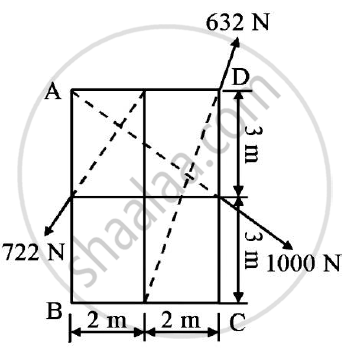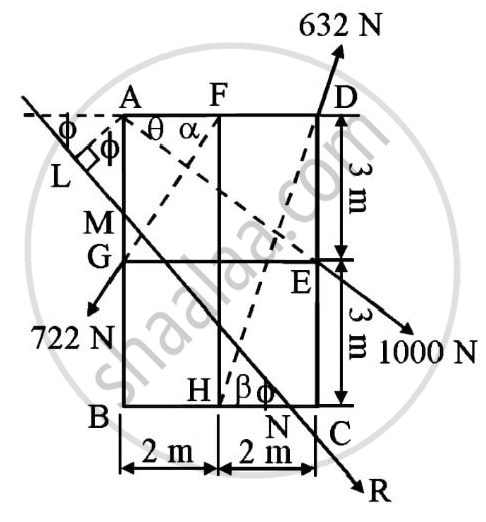Advertisement Remove all ads

# Compute the Resultant of Three Forces Acting on the Plate Shown in the Figure. Locate It’S Intersection with Ab and Bc. - Engineering Mechanics

Answer in Brief

Compute the resultant of three forces acting on the plate shown in the figure. Locate it’s intersection with AB and BC.Advertisement Remove all ads

#### Solution

Solution :
Given : Various forces acting on a body
To find : Resultant of the forces and intersection of resultant with AB and BC
Solution :In △ AFG ,
tanα = (AG)/(AF) =(DE)/(BH) = 3/2 = 1.5
α = tan-1(1.5) = 56.31°
In △DAE,

tan θ = (DE)/(AD)=(DE)/(BC) =3/4 = 0.75
θ = tan-10.75 = 36.87°

In △DHC
tanβ =(DC)/(HC) =6/2 = 3
β = tan-1(3)
β = 71.565°

Assume R be the resultant of the forces
ΣFx = -722cos α + 1000cos θ + 632cos β
= 599.3624 N
ΣFy = -722sin α - 1000sin θ + 632sin β
= -601.1725 N
R=sqrt((ΣFx)^2+(ΣFy)^2)
R=sqrt((599.3624)^2+(−601.1725)^2
R=848.9073 N

ϕ = tan-1(Σ Fy)/(ΣFx)
= tan-1 ((-601.1725)/(599.3624))
= 45.0863° (in fourth quadrant)
Let R cut AB and BC at points M and N respectively
Draw AL ⊥ R

Taking moments about point A
MA = 632 sin β x AD -722cos α x AG
= 632 x sin71.565o x 4 – 722cos56.31° x 3
=1196.7908 Nm
Applying Varigon’s theorem
MA = R x AL
1196.7908 = 848.9073 x AL
AL=1.4098 m
In △AML,
cos Ф = (AL)/(AM)
cos 45.0863 =(1.4098)/(AM)
AM = 1.9967 m
MB = AB - AM
= 6 - 1.9967
= 4.0033 m
In △BMN
tan Ф =(BM)/(BN)
tan 45.0863=(4.0033)/(BN)
R=848.9073 N (45.0863° in fourth quadrant)
Resultant force intersects AB and BC at M and N such that AM=1.9967 m and BN=3.9912 m

Concept: Velocity Diagrams for Bodies in Plane Motion.
Is there an error in this question or solution?
Advertisement Remove all ads

#### APPEARS IN

Advertisement Remove all ads
Advertisement Remove all ads
Share
Notifications

View all notifications

Forgot password?